# How to Count Occurrences in Entire Workbook in Excel

This post will guide you how to count number of occurrences in whole workbook in Excel 2013/2016 or Excel office 365. How do I count how many times a string is repeated in all worksheets in Excel. You may be want to count occurrences for the number of times a certain value matches across all worksheets in your current workbook. And you can do this by a formula based on the SUMPRODUCT  function, the COUNTIF function and the INDIRECT function in Excel.

General Formula:

The below general formula can help you to count occurrences in a certain range across the entire workbook in Excel. Like this:

=SUMPRODUCT(COUNTIF(INDIRECT(“‘”&mysheets&”‘!”&range),criteria))

Note: you need to put the names of your all worksheets(sheet1,sheet2,sheet3) into cells. And then select this range, clicking on the drop-down arrow along the formula bar and name this range as “mysheets”.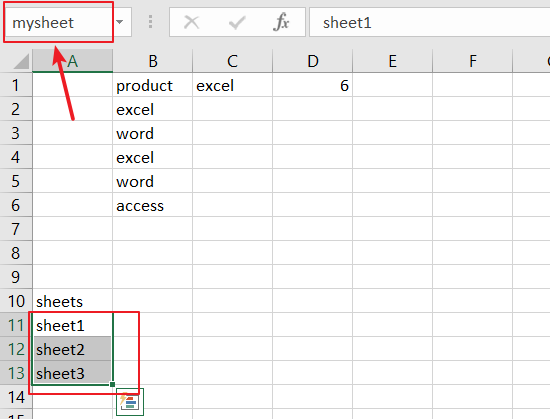## Count Occurrences in Entire Workbook

Assuming that you have a data of list in your workbook, and this workbook has three worksheets. And those three worksheets are “Sheet1”,”Sheet2” and “Sheet3”that contain name lists in range B2:B6. And you want to count the number of occurrences of the product name “excel” within a specified range B2;B6 across those three worksheets. And you can use a formula based on the SUMPRODUCT function, the COUNTIF function and the INDIRECT function. Like this:

=SUMPRODUCT(COUNTIF(INDIRECT(“‘”&mysheet&”‘!B2:B6”),C1))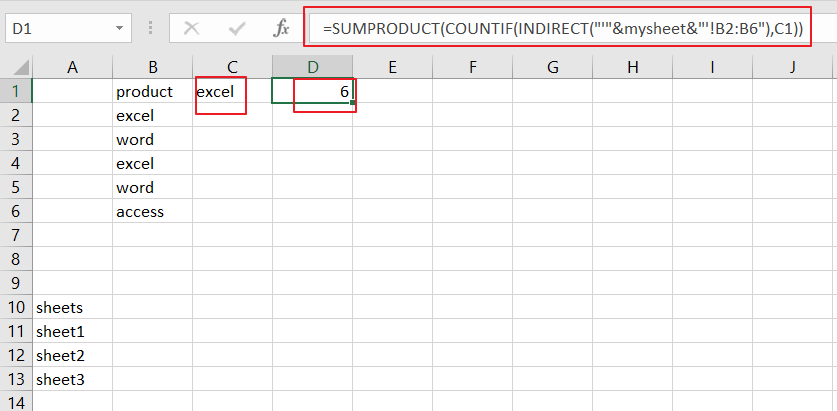Let’s See That How This Formula Works:

=”‘”&mysheet&”‘!B2:B6″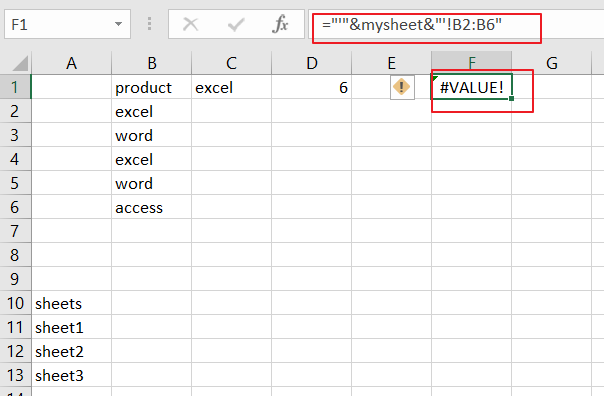You know that mysheet is a named range that contains “sheet1”,”sheet2” and “sheet3”. This formula will return an array result like this:

{“‘sheet1’!B2:B6″;”‘sheet2’!B2:B6″;”‘sheet3’!B2:B6”}=INDIRECT(“‘”&mysheet&”‘!B2:B6”)

The INDIRECT function can be used to convert each text value to a cell reference.

=COUNTIF(INDIRECT(“‘”&mysheet&”‘!B2:B6”),C1)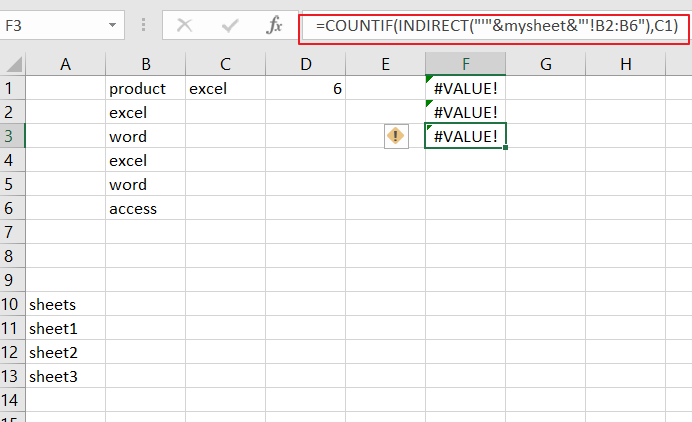The COUNTIF function can be used to count the number of occurrences the value(cell c1) in those range B2:B6 match the value “excel”. And it also returns an array result contains count values from each worksheet.

{2;2;2}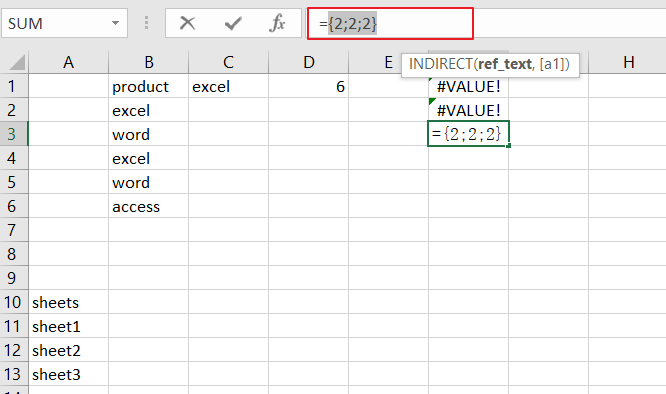Finally, the SUMPRODUCT function sums the above array results returned by the COUNTIF function.

### Related Functions

• Excel SUMPRODUCT function
The Excel SUMPRODUCT function multiplies corresponding components in the given one or more arrays or ranges, and returns the sum of those products.The syntax of the SUMPRODUCT function is as below:= SUMPRODUCT (array1,[array2],…)…
• Excel INDIRECT function
The Excel INDIRECT function returns the cell reference based on a text string, such as: type the text string “A2” in B1 cell, it just a text string, so you can use INDIRECT function to convert text string as cell reference….
• Excel COUNTIF function
The Excel COUNTIF function will count the number of cells in a range that meet a given criteria. This function can be used to count the different kinds of cells with number, date, text values, blank, non-blanks, or containing specific characters.etc.= COUNTIF (range, criteria)…